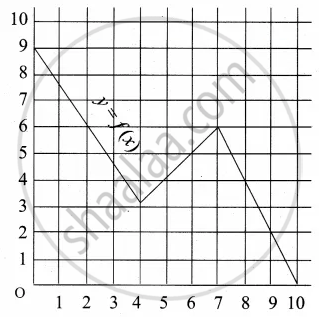Tamil Nadu Board of Secondary EducationSSLC (English Medium) Class 10th

# A graph representing the function f(x) is given in it is clear that f(9) = 2 Find the following values of the function (a) f(0) (b) f(7) (c) f(2) (d) f(10) - Mathematics

Graph
Sum

A graph representing the function f(x) is given in it is clear that f(9) = 2Find the following values of the function

(a) f(0)

(b) f(7)

(c) f(2)

(d) f(10)

#### Solution

(a) f(0) = 9

(b) f(7) = 6

(c) f(2) = 6

(d) f(10) = 0

Concept: Functions
Is there an error in this question or solution?